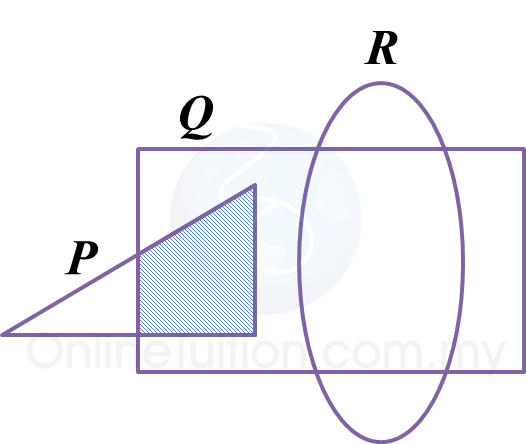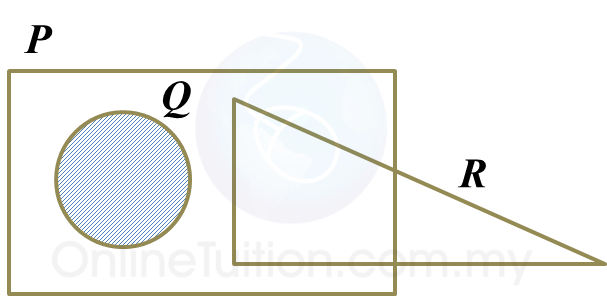# 4.5.3 Operations on Sets, SPM Paper 2 (Long Questions)

Question 5:
The Venn diagrams in the answer space shows sets P, Q and R such that the universal set, $\xi =P\cup Q\cup R$
(a) P Q,
(b) $Q\cap \left({P}^{‘}\cup R\right).$Solution:
(a)
P Q(b)
$Q\cap \left({P}^{‘}\cup R\right).$Question 6:
The Venn diagrams in the answer space shows sets P, Q and R such that the universal set, $\xi =P\cup Q\cup R$
(b) $P\cap {\left(Q\cup R\right)}^{‘}.$$P\cap {\left(Q\cup R\right)}^{‘}.$